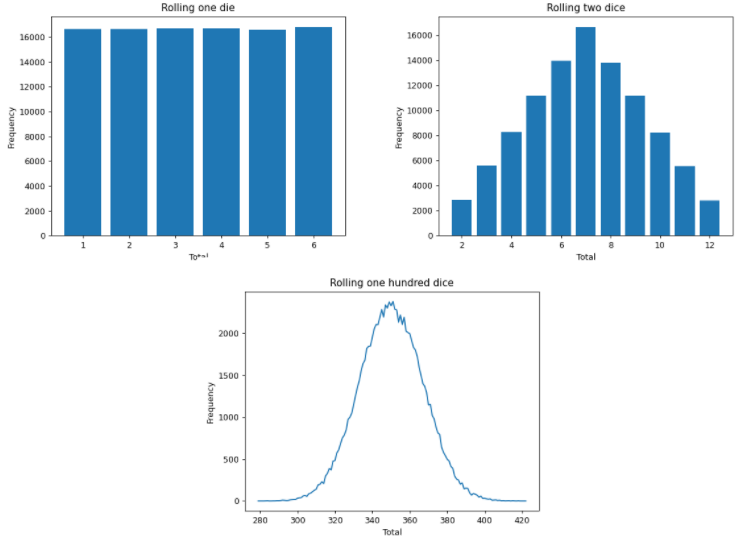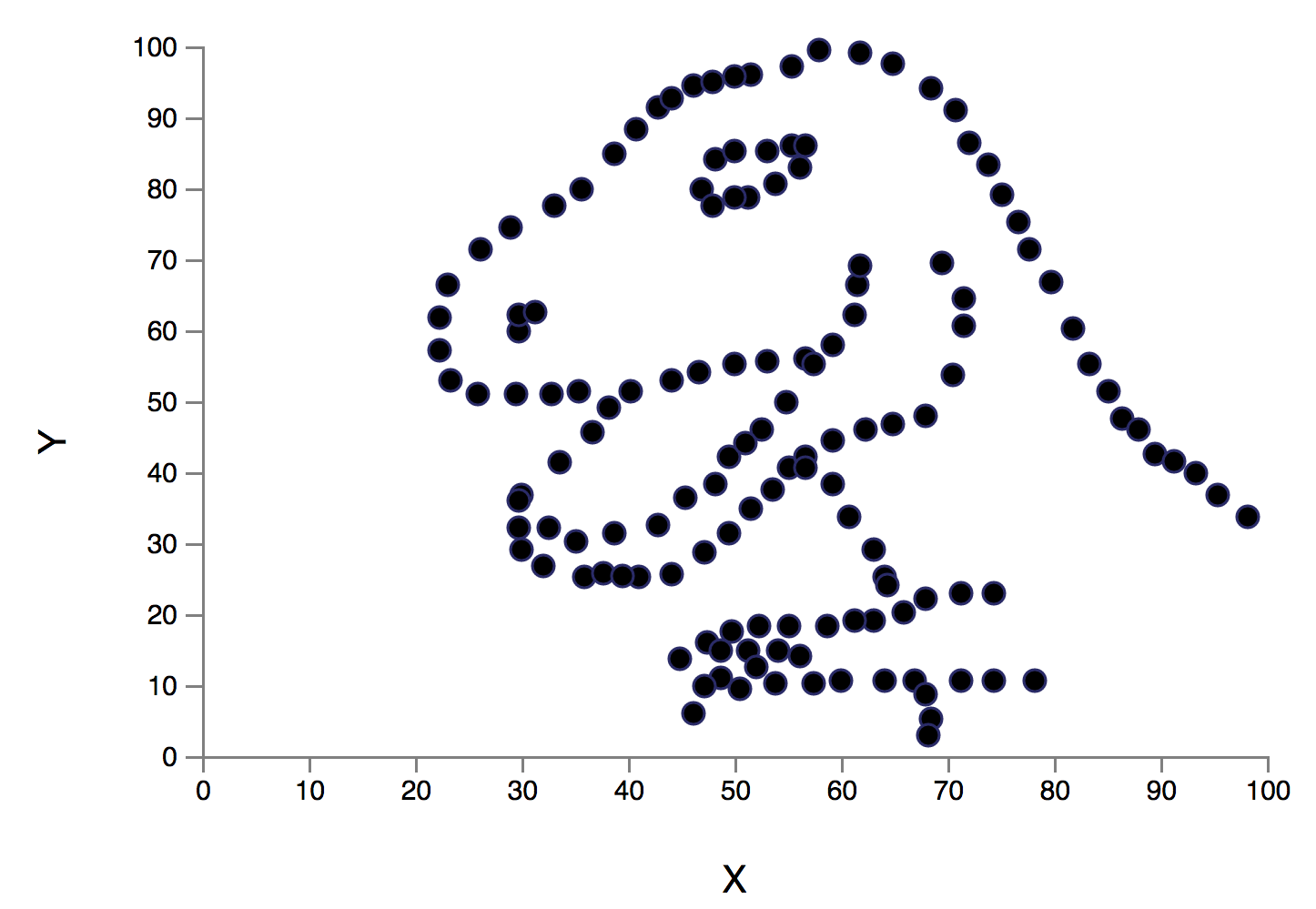More than the mean: the importance of graphing

By Michael Conterio. Posted

Anscombe’s quartet: all four sets of data share the same set of statistical measures, but plot very differently on graphs

Using statistical measures such as the mean is important for understanding data — but they can hide just as much as they reveal

The average planet in our solar system has zero, zero, or about a billion people on it, depending on whether you take the median, mode, or mean. Obviously none of these figures quite captures the real situation, with all of the population living on (or just above, in the case of the International Space Station) just one of the eight planets — and nor does calculating the range or the standard deviation. What else could these statistical measures be hiding, that could be uncovered by drawing a graph?

Much of the time, using averages and measures of spread such as the interquartile range and standard deviation can be useful for getting a quick feel for a set of data, or to easily compare two different sets of data. Whether it’s results between different classes in your school, medical outcomes, or tests being run on different prototypes of a new product, if two sets of data have different means and standard deviations, you can use these to describe some of the key differences between those sets.

However, some of your students may think that the definite and exact values they get from their calculations are more important than something as open to interpretation as the shape of a graph. Luckily, the real world provides examples of collections of data that behave very differently, which becomes obvious when you graph your data.

Finding the shape of data

An activity you might use in your classes when looking at data and statistics is to look at the outcomes of rolling a fair standard six-sided die. In this case, there’s an equal chance of rolling any of the numbers, leading to a flat distribution if you graph it. The mean and median values of a large number of dice rolls will be 3.5, while there isn’t a single modal value.Start looking at the rolls of a pair of dice, however, and the graph changes shape significantly. Although the mean doubles to 7, the graph is no longer flat; there is a peak at 7 and the chances of rolling each value decreases as you move away from this, towards 2 or 12. As you increase the number of dice you are rolling at once, the graph moves towards a commonly observed shape, a curve known as the normal/Gaussian distribution, or bell curve. You can find this same shape right across the sciences, whether you are looking at how far molecules have diffused after a given time, the sizes of animals, or random errors in an experiment. It’s important for everyone to appreciate the long tails of these graphs — even though most of the data will fit inside the main body of the curve, there’s plenty of space further away where you might find a rarer specimen.

Unusual shapes can also help you to spot interesting areas to study — or at least to tidy up your data. Sets of data can often include outliers, points that don’t seem to fit with the rest of the distribution, and drawing a graph of your data can help you to spot these. These could be due to experimental error, or they could demonstrate an unusual or rare effect. Either way, these outliers could be worth investigating, even if the end result is that you remove them so that your remaining data more accurately represents what you are investigating.

Same stats, different graphs

As well as hiding the details of a data set, just using statistical measures can also hide the differences between different data sets, a fact which has been well known to teachers of statistics for a while. In 1973 the statistician Francis Anscombe created four sets of data that clearly appear different when plotted on scatter diagrams, but share the same set of statistical measures, including the means and standard deviations of both x and y.

The first two graphs (see image at the top of the page) show common shapes on scatter plots. The straight line shows a linear relationship between two variables: as you increase one, the other increases by a proportional amount. The second shows a slightly more complicated but still common relationship: a quadratic relationship. Although these graphs are clearly distinct, they share the same mean values and standard deviations.

The other two graphs show how much an outlier can affect your interpretation of the data. If you just use the statistical measures, these sets of data would look the same as each other, as well as the previous two.

In fact, with some careful manipulation you can create a whole set of graphs that share the same statistics, but look very different to each other — even as far as giving a picture of a dinosaur with the same statistics as a set of rings (see image and helloworld.cc/datasaurus).You could use these data sets to really emphasise this to your students. You might present the statistical values and ask students to draw what they think the graphs would look like, before revealing the range of possible graphs. Or you might give each student a data set and the corresponding graph, asking them to calculate the x- or y-mean, before bringing the class back together to share the results that they’ve all calculated the same values. Either way, this could help start a discussion about why graphs are useful.

Summarising data

I hope I’ve convinced you about the importance of encouraging your students to use graphs to look beyond the mean and think more about the shape of data. I suggest that you make the time to plot and look at graphs, and ask your students to describe in words what the graphs they’ve plotted tell us. And maybe then you can link it all back to the statistical measures we’ve been avoiding so far — and if they can link their numerical calculation with the graphs, they might gain a better grasp of just what the mean means.Print

Free - UK only

If you’re a UK-based teacher, volunteer, librarian or something in between, we'll send each issue free to your door.

Digital

Free

Just want to read the free PDF? Get each new issue delivered straight to your inbox. No fuss and no spam.